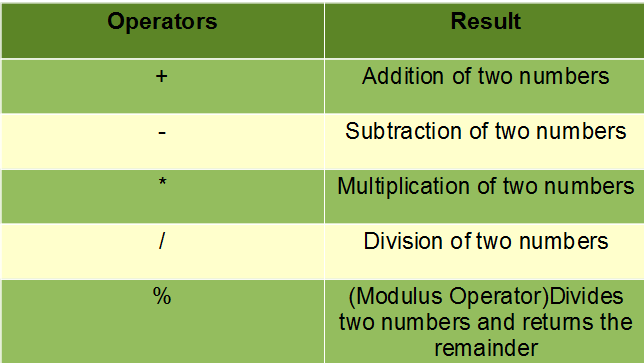Open in App
Not now

## Related Articles

• Write an Interview Experience

# Java Arithmetic Operators with Examples

• Difficulty Level : Easy
• Last Updated : 06 Dec, 2021

Operators constitute the basic building block to any programming language. Java too provides many types of operators which can be used according to the need to perform various calculations and functions, be it logical, arithmetic, relational, etc. They are classified based on the functionality they provide. Here are a few types:

This article explains all that one needs to know regarding Arithmetic Operators.

### Arithmetic Operators

These operators involve the mathematical operators that can be used to perform various simple or advanced arithmetic operations on the primitive data types referred to as the operands. These operators consist of various unary and binary operators that can be applied on a single or two operands. Let’s look at the various operators that Java has to provide under the arithmetic operators.Now let’s look at each one of the arithmetic operators in Java:

1. Addition(+): This operator is a binary operator and is used to add two operands.

Syntax:

`num1 + num2`

Example:

```num1 = 10, num2 = 20
sum = num1 + num2 = 30```

## Java

 `// Java code to illustrate Addition operator`` ` `import` `java.io.*;`` ` `class` `Addition {``    ``public` `static` `void` `main(String[] args)``    ``{``        ``// initializing variables``        ``int` `num1 = ``10``, num2 = ``20``, sum = ``0``;`` ` `        ``// Displaying num1 and num2``        ``System.out.println(``"num1 = "` `+ num1);``        ``System.out.println(``"num2 = "` `+ num2);`` ` `        ``// adding num1 and num2``        ``sum = num1 + num2;``        ``System.out.println(``"The sum = "` `+ sum);``    ``}``}`

Output

```num1 = 10
num2 = 20
The sum = 30```

2. Subtraction(-): This operator is a binary operator and is used to subtract two operands.

Syntax:

`num1 - num2`

Example:

```num1 = 20, num2 = 10
sub = num1 - num2 = 10```

## Java

 `// Java code to illustrate Subtraction operator`` ` `import` `java.io.*;`` ` `class` `Subtraction {``    ``public` `static` `void` `main(String[] args)``    ``{``        ``// initializing variables``        ``int` `num1 = ``20``, num2 = ``10``, sub = ``0``;`` ` `        ``// Displaying num1 and num2``        ``System.out.println(``"num1 = "` `+ num1);``        ``System.out.println(``"num2 = "` `+ num2);`` ` `        ``// subtracting num1 and num2``        ``sub = num1 - num2;``        ``System.out.println(``"Subtraction = "` `+ sub);``    ``}``}`

Output

```num1 = 20
num2 = 10
Subtraction = 10```

3. Multiplication(*): This operator is a binary operator and is used to multiply two operands.

Syntax:

`num1 * num2`

Example:

```num1 = 20, num2 = 10
mult = num1 * num2 = 200```

## Java

 `// Java code to illustrate Multiplication operator`` ` `import` `java.io.*;`` ` `class` `Multiplication {``    ``public` `static` `void` `main(String[] args)``    ``{``        ``// initializing variables``        ``int` `num1 = ``20``, num2 = ``10``, mult = ``0``;`` ` `        ``// Displaying num1 and num2``        ``System.out.println(``"num1 = "` `+ num1);``        ``System.out.println(``"num2 = "` `+ num2);`` ` `        ``// Multiplying num1 and num2``        ``mult = num1 * num2;``        ``System.out.println(``"Multiplication = "` `+ mult);``    ``}``}`

Output

```num1 = 20
num2 = 10
Multiplication = 200```

4. Division(/): This is a binary operator that is used to divide the first operand(dividend) by the second operand(divisor) and give the quotient as a result.

Syntax:

`num1 / num2`

Example:

```num1 = 20, num2 = 10
div = num1 / num2 = 2```

## Java

 `// Java code to illustrate Division operator`` ` `import` `java.io.*;`` ` `class` `Division {``    ``public` `static` `void` `main(String[] args)``    ``{``        ``// initializing variables``        ``int` `num1 = ``20``, num2 = ``10``, div = ``0``;`` ` `        ``// Displaying num1 and num2``        ``System.out.println(``"num1 = "` `+ num1);``        ``System.out.println(``"num2 = "` `+ num2);`` ` `        ``// Dividing num1 and num2``        ``div = num1 / num2;``        ``System.out.println(``"Division = "` `+ div);``    ``}``}`

Output

```num1 = 20
num2 = 10
Division = 2```

5. Modulus(%): This is a binary operator that is used to return the remainder when the first operand(dividend) is divided by the second operand(divisor).

Syntax:

`num1 % num2`

Example:

```num1 = 5, num2 = 2
mod = num1 % num2 = 1```

## Java

 `// Java code to illustrate Modulus operator`` ` `import` `java.io.*;`` ` `class` `Modulus {``    ``public` `static` `void` `main(String[] args)``    ``{``        ``// initializing variables``        ``int` `num1 = ``5``, num2 = ``2``, mod = ``0``;`` ` `        ``// Displaying num1 and num2``        ``System.out.println(``"num1 = "` `+ num1);``        ``System.out.println(``"num2 = "` `+ num2);`` ` `        ``// Remaindering num1 and num2``        ``mod = num1 % num2;``        ``System.out.println(``"Remainder = "` `+ mod);``    ``}``}`

Output

```num1 = 5
num2 = 2
Remainder = 1```

My Personal Notes arrow_drop_up